# Dynamics analysis on barrel considering the temporal and spatial distribution of propellant gas by numerical simulation

## Qingbo Yu1, Guolai Yang2, Quanzhao Sun3

1, 2, 3School of Mechanical Engineering, Nanjing University of Science and Technology, Nanjing, 210094, China

2Corresponding author

Journal of Vibroengineering, Vol. 20, Issue 4, 2018, p. 1588-1602. https://doi.org/10.21595/jve.2018.19623
Received 18 May 2017; received in revised form 2 January 2018; accepted 9 January 2018; published 30 June 2018

Copyright © 2018 Qingbo Yu, et al. This is an open access article distributed under the Creative Commons Attribution License, which permits unrestricted use, distribution, and reproduction in any medium, provided the original work is properly cited.
Views 849
Abstract.

Highlights
• Provide a convenient method of modeling the gas pressure loading process with the assistance of user-defined subroutines in ABAQUS program
• The consideration of the radial effect of propellant gas stimulates more pronounced dynamic results
• Structural inhomogeneity can directly affect the dynamic response and brings more pronounced results

#### 1. Introduction

Firing accuracy of large-caliber artillery has become a focused issue. When a weapon is firing, there are vibrations induced in the steel barrel as the bullet moves along it. The interaction of projectile with barrel and dynamic loading process of propellant gases are primary factors for motivating barrel vibration. Propellant gases pressure belongs to high-impact load and its amplitude and loading distribution vary with time, characterized with dynamic effect of step type pulse and playing a non-neglected role in motivating barrel vibration. In consideration of the interaction of barrel with projectile, the vibration of barrel acts as the excitation factor for the movement of projectile during interior ballistics process . Most researchers work at firing accuracy from the point of view of barrel vibration and have confirmed that the muzzle initial disturbance is the key factor that affects the projectile dispersion . Reference  treated the barrel as a cantilever beam that can recoil freely and formed the moving mass-cantilever beam system together with the projectile and the inertial breech. The Bernoulli-Euler beam model was used for describing that system to study the vibrational response of the barrel of a rarefaction wave gun by numerical analysis. Reference  established the transverse vibration equation of a barrel considering the inertia effect of projectile to investigate the vibration characteristics of barrel and numerically simulated the vibration characteristics of barrel with different moving parameters. Reference  set up the time-variant dynamic equations of the tube radial vibration subjected to a moving projectile by Euler-Bernoulli beam theory. The tube vibration of small-caliber guns was studied when projectile moving along a rifling with finite element method and cantilever beam theory. In those works, The Bernoulli-Euler beam model was adopted to approximately substitute the actual interaction between barrel and projectile. Nevertheless, that model is only an idealized physical substitution and cannot consider the real physical mechanism of firing process. On the other hand, the radical effect of propellant gas was ignored, which inevitably causes the deviation of the computational results from the experimental ones.

Numerical simulation with the aid of convenient modeling platform and efficient computation method has become a significant tool to simulate artillery firing process. Numerical simulation have excellent numeric, visible, and reappearance attributes that can help engineers design and develop a new system efficiently and has already become an essential tool in contending with instantaneous reaction phenomenon analyses, such as those encountered during firing cycles . Reference  developed a three dimensional finite element model of a large caliber gun using ANSYS commercial software and simulated its firing process in order to study the effects of various design factors on the dynamics response of the gun. Reference  modeled both the barrel and bullet by LS-DYNA software and investigated the influence of the barrel fixing on barrel vibration when a bullet moves down the barrel during shot by numerical simulation. Reference  established a guided projectile-barrel coupled dynamic finite element model based on elastic and plastic finite element theory and studied the projectile-barrel coupled system of large caliber gun for launching a guided projectile with sliding driving band. Most of the existing researches on the movement of projectile in bore and the vibration response of barrel during firing cycle focused on the interaction of barrel with projectile, ignoring the radial effect of propellant gas acting on the inner wall of the barrel.

This paper is aimed to involve the radial effect of propellant gas while analyzing barrel dynamics response by numerical simulation. The coupling process between projectile motion and propellant combustion was translated by classical interior ballistic equations with VUAMP subroutine, which produces loading boundary condition for simulation model. The dynamic loading process of gas pressure on barrel was modeled by the VDLOAD subroutine. The dynamics response with and without the radial effect of gas pressure being considered were compared, and the effect of mass eccentricity of barrel was also studied.

#### 2. Mechanical model

Referring to the Euler-Bernoulli beam model , the barrel can be simplified as a variable cross-section cantilever, one end of which being fixed and the other end being free. The barrel suffers from the comprehensive function of the expansion of gunpowder gas and the collision with the projectile throughout the interior ballistic process. The simple mechanical model is illustrated in Fig. 1. In the figure, $M$ is the mass of the projectile. ${F}_{cont}$ is the equivalent force from the motion of projectile irrespective of the inertia effect applied to the barrel. ${v}_{t}$ is the movement speed of projectile at corresponding time. The space past projectile is where gunpowder gas function, and the loading condition of gunpowder gas on the inner wall of the barrel changes dynamically with the movement of projectile, which belongs to the time-varying dynamic system.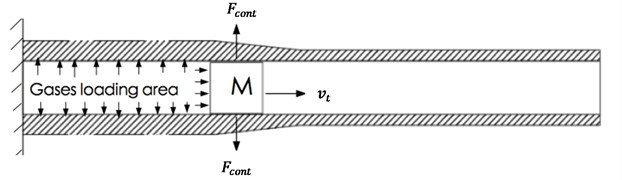According to the D’Alembert principle , the tube radial motion equation under the function of moving projectile and gunpowder gas pressure can be described as Eq. (1), which takes into account of the damping and projectile inertial effects:

(1)
$\frac{{\partial }^{2}}{\partial {x}^{2}}\left[EI\left(x\right)\frac{{\partial }^{2}}{\partial {x}^{2}}y\left(x,t\right)\right]+\rho A\left(x\right)\left[\frac{{\partial }^{2}}{\partial {t}^{2}}y\left(x,t\right)+2H\frac{\partial }{\partial t}y\left(x,t\right)\right]=F,$

where $EI\left(x\right)$ represents the flexural stiffness of barrel, $H$ is speed attenuation coefficient of barrel, and $y\left(x,t\right)$ represents the deflection of barrel corresponding to time and location. $F$ represents the loading of barrel, and can be formulated as Eq. (2):

(2)
$F=\delta \left(x-s\left(t\right)\right){F}_{cont}+\sum \xi \left(x-s\left(t\right)\right)P\left(x,t\right)ds,$
(3)
${F}_{cont}=F\left(t\right)+M\left(\frac{{\partial }^{2}y}{\partial {t}^{2}}+2v\frac{{\partial }^{2}y}{\partial t\partial x}+{v}^{2}\frac{{\partial }^{2}y}{\partial {x}^{2}}\right),$

where $\delta$ is the Dirac function; $s\left(t\right)$ is the axial displacement of projectile at corresponding time: $s\left(t\right)=\int {v}_{t}dt$. ${F}_{cont}$ is the force acting on the inner wall considering inertial effect of projectile, and $F\left(t\right)$ corresponds to the condition without inertial effect of projectile. The right part of Eq. (3) is the additional items when taking into account of the inertia effect of projectile. In order to express the dynamic loading condition of powder gases varying with projectile motion, the function $\xi \left(x\right)$ was defined as Eq. (4):

(4)
$\xi \left(x\right)=\left\{\begin{array}{l}1,\begin{array}{ll}& x\le 0,\end{array}\\ 0,\begin{array}{ll}& \text{else}\end{array}.\end{array}\right\$

In order to simulate the real loading conditions that the barrel suffers and investigate the effect of propellant gas on the dynamics response of barrel, the physical structure can be discretized by finite element analyses software to approximately replace the actual characteristics. The central difference method can be applied to compute corresponding mechanical model.

#### 3. Numerical simulation

In this paper, the projectile-barrel coupling system for a large caliber of 122-mm howitzer was taken as the research object. The dynamics response during interior ballistic process was numerically simulated allowing for the radial effect of propellant gas.

#### 3.1. Finite element modeling

With the aid of preprocessing software HYPERMESH, the coupling model of projectile and barrel was discretized with enough rationality and accuracy. As the barrel contains mixed rifles with large spiral angle and the structure is complicated, meshes were established from the origination of rifling, and the FE model of barrel was obtained by scanning stretching along the space curve of rifling mostly with eight-node hexahedron element (C3D8R). The local view of the forcing cone is shown in Fig. 2.

According to the actual structure size and shape characteristics, the projectile was discretized with reasonable simplifications and assumptions, mainly with eight-node hexahedron element. The corresponding motion parameters such as mass, mass center position and moment of inertia remained consistent with actual condition. The projectile body was assumed to be rigid and the copper band was considered as elastic-plastic. The dynamic process also neglects the effect of rotating band engraving into bore process. The reserved grave on the rotating band matching the rifling shape was preset and the instant when rotating band completely engraves into bore was set as the initial time. The discretization model of projectile with preset groove is shown in Fig. 3. The whole coupling model has globally 651572 solid elements and 548380 nodes.

Node-surface contact was established to define the interaction between rotating band and barrel, and the interaction between front bourrelet and barrel was defined by surface-to surface contact. The complicated condition of boundary non-linearity could bring difficulty to computation convergence. Penalty function method, as a result of its accurate momentum conservation and rare generation of the hourglass effect, is adopted to deal with contact interface constraints. In each increment step, the slave nodes are checked whether to penetrate the master surface to ensure that the mutual penetration will not occur between contact surfaces. When the penetration takes place, the contact force proportional to the magnitude of penetration is introduced into the contact surface.

Fig. 2. Local view of the forcing cone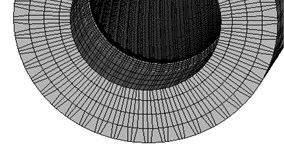Fig. 3. The discretization model of projectile with preset groove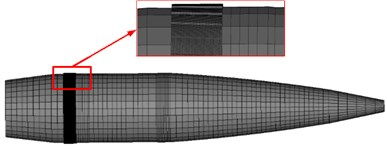#### 3.2. Nonlinear constitutive model

When a large-caliber gun fires, the duration of the projectile in gun bore is generally a few microseconds. The complicated physics fields during IB process makes rotating band subjected to the comprehensive environment of large strain, high temperature and high strain rate. Copper band exhibits liable deformation capacity, and the establishment of reasonable elastic-plastic constitutive model is the key to the simulation analysis. A modified Johnson-Cook model calibrated by Ma  was introduced to describe the mechanical behavior in plastic deformation phase. The associated constitutive model can be defined by Eq. (5):

(5)
$\stackrel{-}{\sigma }=\left[A+B{\left({\epsilon }^{p}\right)}^{n}\right]\left(1+C\mathrm{l}\mathrm{n}\stackrel{˙}{\epsilon }\right)\left[1-{\left({T}^{*}\right)}^{m}\right],$

where $\stackrel{-}{\sigma }$ is flow stress of material. $A$ is yield strength under quasi-static test. $B$ and $n$ are material strain-hardening parameters. ${\epsilon }^{p}$ is equivalent plastic strain. $c$ is strain rate dependent coefficient. $\stackrel{˙}{\epsilon }$ is plastic strain rate. $m$ is temperature softening coefficient. ${T}^{*}$ is relative temperature:

(6)
${T}^{*}=\frac{T-{T}_{r}}{T-{T}_{m}},$

where${T}_{r}$ represents the reference temperature, which was set as an ambient temperature. ${T}_{m}$ is the melting temperature of the material under normal condition.

The barrel is made of special steel PCrNi3MoVA, and rotating band is made of brass H96. The associated parameters are listed in Table 1.

Table 1. Material parameters for numerical computation

 Material Density / Kg·m-3 Poisson’s ratio Elastic modulus / MPa Barrel Gun-steel 7900 0.3 205000 Band Brass 8400 0.35 118000 A/MPa B/MPa C $m$ $n$ 90 292 0.025 0.31 1.09

#### 3.3. The implementation of changing physics fields

Artillery interior ballistic process is characterized with complicated variation of physical fields, mainly reflected by the changes of gunpowder gas pressure and the changing loading area of tube, namely the temporal and spatial distribution characteristics. Gunpowder gas flows along the axial direction of tube, propelling the projectile to complete interior ballistic movement. On the other hand, gunpowder gas pressure acts on the inner wall of tube, and the pressure value and loading area vary dynamically with the movement of projectile. The pressure spatial distribution of gas pressure can be realized according to the position of projectile at certain moment. Using VUAMP and VDLOAD subroutine interfaces can accomplish the physical fields changing process during interior ballistic process .

#### 3.3.1. The implementation of gunpowder combustion process

As the pressure load is generated from propellant combustion and will act at the bottom of the projectile. Some researchers at present often utilize modified pressure (based on the testing chamber pressure) in the simulation. The frictional work and the plastic work will be taken into account twice and incorrect results are to be obtained. Therefore, User subroutine VUAMP is implemented within the FE model in order to take account of the coupling effect of powder combustion and projectile movement with the purpose of computing the appropriate base pressure of projectile. Some assumptions have been done beforehand for interior ballistic coupling course:

(a) Ignore the projectile’s seizing-bore and band engraving into bore process at the initial stage of interior ballistic process. Assume that the average pressure at the instant when band completely engraves into bore is 30 MPa, set as the initial value of the chamber pressure.

(b) The center point of the bore bottom is set as the origin of the coordinate system, and the $x$ axis is defined by the axis of barrel pointing to muzzle. The gas pressure distribution based on the Lagrangian model assumption is referred to define the distribution of gases pressure on the inner wall of barrel, which demonstrates parabolic distribution shown as Eq. (7):

(7)
$P（x,t）=\frac{3\phi m}{w+3\phi m}{P}_{t}\left[1+\frac{w}{2\phi m}\left(1-\frac{{x}^{2}}{{{s}_{t}}^{2}}\right)\right],$

where ${s}_{t}$ is movement displacement of projectile, $\phi$ is second power coefficient, and ${P}_{t}$ represents average gases pressure.

Based on Eq. (7), the pressure at the base of projectile can be defined as the function of mean pressure expressed as Eq. (8):

(8)
${P}_{d}=\frac{3\phi m}{w+3\phi m}{P}_{t}.$

Identically, the pressure at the base of chamber is defined as Eq. (9):

(9)
${P}_{\mathrm{t}\mathrm{a}\mathrm{n}g}=\frac{3\phi m}{w+3\phi m}{P}_{t}\left(1+\frac{w}{2\phi m}\right).$

Fortran language was utilized to compile the propellant combustion process. At each computation increment, numerical computation model transfer movement qualities of projectile in time, by which subroutine program generate inner-bore mean pressure ${P}_{t}$ according to predefined powder combustion rule. The result was transferred to main program in return. The pressure at the bottom of projectile was obtained based on Eq. (8), which drives the projectile moving towards muzzle.

The computation model utilizes module charge, and the corresponding classical IB equations can be presented as follows:

(10)
$\left\{\begin{array}{l}\frac{dz}{dt}=\frac{{u}_{1}}{{e}_{1}}{p}^{n},\\ \psi =\frac{{w}_{1}{\psi }_{1}+{w}_{2}{\psi }_{2}}{w}={\alpha }_{1}{\psi }_{1}+{\alpha }_{2}{\psi }_{2},\\ {\psi }_{1}={\chi }_{1}{z}_{1}\left(1+{\lambda }_{1}{z}_{1}+{\mu }_{1}{{z}_{1}}^{2}\right),\\ {\psi }_{2}={\chi }_{2}{z}_{2}\left(1+{\lambda }_{2}{z}_{2}+{\mu }_{2}{{z}_{2}}^{2}\right),\\ p=\frac{fw\psi -\frac{\theta }{2}\phi m{v}^{2}}{S\left({l}_{0}+l\left(t\right)\right)},\end{array}\right\$

where $Z$ is the propellant relative burned thickness. $\psi$ is the relative burned volume. $\chi$, $\lambda$ and $\mu$ are IB parameters related to propellant shape. $w$ is the mass of propellant, $f$ is the propellant impetus, ${l}_{0}$, $l\left(t\right)$ are equivalent length of chamber and displacement of projectile, respectively, ${u}_{1}$ is the constant of burning rate, ${e}_{1}$ is the half thickness of the multiple-perforated propellant, $n$ is the pressure index of burning rate, $\theta$ is thermal index, $S$ is the sectional area of bore.

Propellant gas pressure acts on the in-wall of barrel, and the loading area varies dynamically with the movement of projectile. Using VDLOAD subroutine can realize actual loading condition of barrel. At each time increment, the main program call subroutine program to do corresponding computation. The basic implementation procedures by VDLOAD subroutine are briefly illustrated as follows:

Step 1: Main program transmit the displacement of projectile and mean gas pressure at real time to subroutine program.

Step 2: All integration points on the in-wall of barrel were preselected as loading units, and subroutine program obtained the coordinate information of all preselected points.

Step 3: Subroutine program conduct judgment analysis and assign pressure values to all points. Each point was assigned values one by one. If the axial coordinate of one point is not more than the displacement of projectile, the value to be assigned is set as the timely gas pressure corresponding to the axial position. On the contrary, the value is zero.

Step 4: If the assignment of values to all points is completed, main program continues computing and enters next increment repeatedly till the end.

Fig. 4. The flow diagram of subroutine operation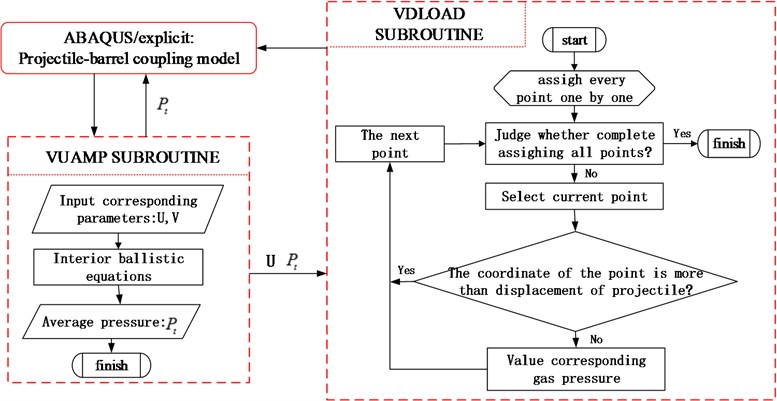The joint use of VUAMP and VDLOAD subroutines realized the coupling process of propellant combustion and movement of projectile and the changing loading condition of barrel. VUAMP subroutine calculate mean gas pressure with the known movement quantities of projectile, which were acquired from main program at certain computation moment. VUAMP subroutine transmits displacement of projectile and mean gas pressure to VDLOAD subroutine to define loading condition of barrel. The entire flow diagram of implementation process by subroutine is shown in Fig. 4.

#### 4. Analyses and results

In the premise of the established numerical model, the central difference method was utilized to perform computations in ABAQUS, a powerful solver widely used in nonlinear dynamics simulation. The average gas pressure was the driving force and was determined by the combustion of gunpowder and the movement of projectile (shown as Eq. (10)), which was implemented with VUAMP subroutine. Simultaneously, the loading process of propellant gas pressure for barrel which is restricted by the motion position was implemented with VDLOAD subroutine. Finally, the computed pressure value and projectile displacement in time domain were recorded and plotted in Fig. 5. The length of projectile in-bore motion is approximately 4030 mm including the length of muzzle brake and the projectile reaches muzzle at the time of 9.88 ms. The driving force at the base of projectile was loaded proportional to the computed pressure in Fig. 5, and the loading condition of barrel was defined according to timely pressure and projectile position. Additionally, the gravity field is considered.

Fig. 5. The time histories of projectile displacement and average pressure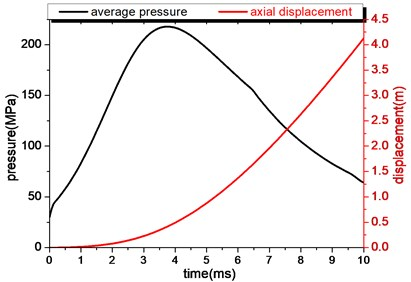Fig. 6 illustrates the corresponding dynamics response of cross-section of barrel at different moment. The rotating band part was hidden in order to highlight the stress distribution of barrel clearly. From the figure, we can clearly see the movement position of projectile in the chamber at different time. The area passed by projectile was occupied as the main stress distribution of barrel, and the load-bearing area of the barrel changes dynamically with the movement of projectile, which is consistent with the actual launch environment. This means that the actual loading condition of gas pressure on the barrel can be considered realized approximately.

Clearly, the stress response of barrel is different at different time. At the time of 5.0 ms, when the pressure is relatively higher, the barrel response is stronger. At any given time, the stress distribution in axial direction is not uniform, which can partly explain the uneven wear of inner wall in axial direction. Furthermore, from the observation of barrel stress distribution, we also find that in the first 3.5 ms the maximum stress is mainly located at forcing cone area, resulting from the higher pressure and thinner structure. Yet in the remaining time, the maximum stress is mainly located at the contact area due to the increasing contact force. This phenomenon can provide valuable references to investigate the dynamic strength of barrel.

Fig. 6. Dynamic change process of stress distribution in axial cross section of barrel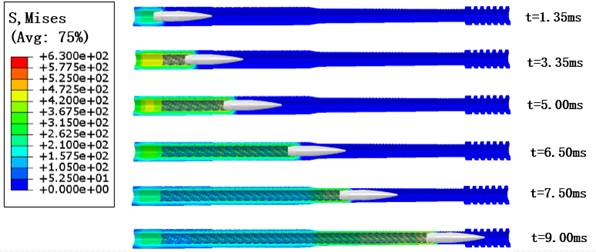#### 4.2. Model verification

The firing trial was conducted under the same shooting conditions with numerical simulation, the obtained muzzle vibration results in the firing trial can be used for comparison to verify the rationality of established model. The angular velocity gyroscope, characterized with small volume, light weight and high reliability, was picked to capture muzzle vibration signal. The obtained signals were then transmitted to data acquisition system for further processing. The installation diagraph of angular velocity gyroscope is shown in Fig. 7, positioned approximately 370 mm from muzzle. The angular velocity gyroscope is SDI-ARG-720, and the data acquisition system is DEWETRON 1201 shown in Fig. 8.

Fig. 7. The installation diagram of angular velocity gyroscope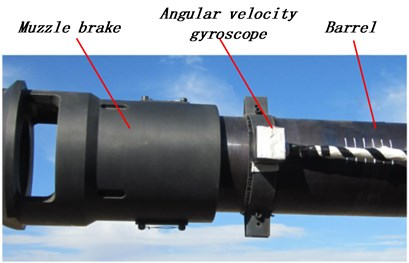Fig. 8. The physics gram of data acquisition system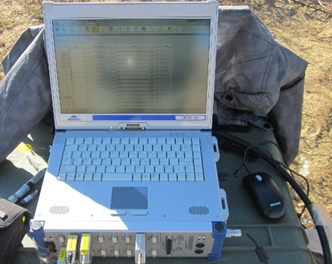Fig. 9. The comparison results of muzzle angular displacement in vertical direction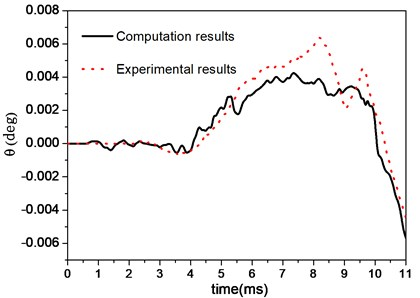Fig. 9 shows the comparison curves of muzzle angular displacement in vertical direction. In spite of slight difference between experimental results and computation results, a similarity in variation trend can be observed. The muzzle vibration values at the in-bore motion completion point are 0.00251 deg and 0.00274 deg, respectively. The relative error is 8.39 %. It implies that the established numerical model can well express the actual physics model.

#### 4.3. The dynamics response of barrel

Propellant gas acts on the barrel directly and causes structure producing some elastic deformation, which affect the vibration of barrel. Aimed at comparison with preceding researches which only considered the interaction between projectile and barrel, two different simulation environments were set in advance, one involving the radial effect of propellant gas and the other only considering the interaction of projectile with barrel.

Fig. 10 and Fig. 11 illustrated the vibration displacement curves in vertical direction and in horizontal direction, respectively. The vibration results reveal that the response of muzzle vibration in the case where the radical effect of propellant gas is considered present more violent than the other case. The initial muzzle vibration in the vertical direction when projectile exit muzzle is 0.548 mm with consideration of radical effect of propellant gas while without the effect it is 0.447 mm, and the same quantity in horizontal direction for two given simulation environments are 0.0139 mm and 0.00274 mm, respectively. This noticeable difference can go back to the actual loading process of barrel and the Space spiral shape of rifling. High pressure gas acts on the in-wall of barrel with Space spiral rifling and this can produce loading dissymmetry, which bring asymmetric elastic deformation of barrel. The above analysis results suggest that the radical effect of propellant gas can cause additional deflection and is essential for studying barrel vibration.

Noticeably, the displacement-time curves in vertical direction under two pre-set simulation environments present similar variation trend like a parabola. This phenomenon can go back to the sustaining action of gravity and cantilever alike structure. The bending deformation of barrel under gravity gives the muzzle point a downward motion tendency. In addition, the projectile dextrorotatory rotation during firing cycle produces a levorotatory torque for the barrel correspondingly. The gravity of the moving projectile causes the contact force to exhibit some inhomogeneity, which also contributes to the drooping trend. For the case when the radial effect of propellant gas is considered, the vibration energy is augmented by gas pressure. Therefore, the magnitudes of two curves present slight difference.

Fig. 10. The muzzle displacement in vertical direction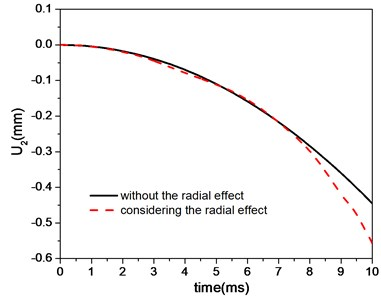Fig. 11. The muzzle displacement in horizontal direction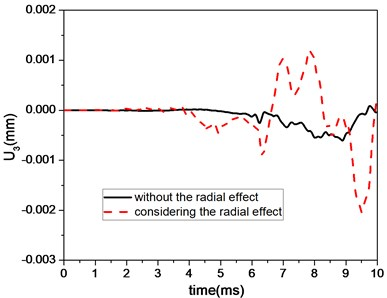The maximum equivalent stress of barrel at different time intervals were recorded and plotted in Fig. 12. For the case when the radical effect of propellant gas is ignored, the max equivalent stress profile shows a progressive increasing trend. It can go back to the increasing contact force with the growth of projectile velocity. Whereas, with the presence of the radical effect of propellant gas, the dynamic strength response is more violent, which can reflect the actual dynamic strength response of barrel more credibly. In the initial phase from the beginning to 5 ms, the difference between the two curves is apparent. Nevertheless, the difference grows small in the following phase when the projectile has already gotten high velocity. This implies that the strength of barrel is directed by gas pressure in the initial phase, while with the growth of velocity, the increasing contact force plays a major role in the strength response. At the end, the magnitudes decline sharply due to the completion of in-bore motion.

For the case when the radial effect of propellant gas was considered, the maximum stress curves in time domain has two distinct peaks with the second peak being higher than the first. The first extreme value is 502 MPa at the approximate time of 3 ms, corresponding to the instant when the gas pressure reaches its extreme point. The second which is also the maximum value during the interior ballistic process reaches 598 MPa, as a result of high inertial force of projectile and the thinner barrel structure. According to the Von Mises yield criterion, the yield stress of barrel material is 1140 MPa, and the safety coefficient can be scaled approximately 2, which identifies the strength requirement of barrel. From the above analyses, we can also conclude that the radical effect of propellant gas is a non-negligible factor for simulating artillery firing process.

Fig. 12. Stress peak distribution curves of barrel in time domain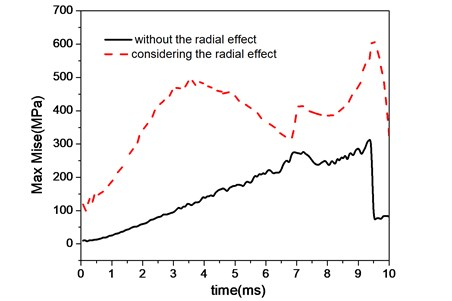#### 4.4. Analysis on the effect of mass eccentricity of barrel on its dynamics response

The present researches involving analysis and modeling of barrel often treated it as the axisymmetric model, and accepted that the cross-section area is uniform. However, the actual manufacturing process cannot guarantee the mass center of barrel precisely located at the central axis. The complicated physical fields acting on the barrel can also cause asymmetrical wear to a certain extent . Furthermore, the material may be redistributed and its mass center maybe deviate from the origin position in vertical direction under the effect of gravity. The different material distribution can influence the overall stiffness and the structural dynamics response. Therefore, it makes more sense to study the dynamics response of barrel considering the existence of mass eccentricity [15, 16]. Nevertheless, due to the complicated loading process of gun barrel and absence of appropriate modeling method, the existent studies often neglect the effect of propellant gas pressure or approximately model the loading process when studying the effect of mass distribution on dynamic response of barrel, which inevitably brings incomplete loading condition and untrustworthy results. With the aid of realization of radial loading of barrel with user subroutine mentioned above, the effect of propellant gas pressure is modeled successfully and the further investigation of the influence of mass distribution on dynamics response of barrel becomes more feasible and convinced.

For the convenience of modeling and calculation, some assumptions were made that the outer contour and the inner contour remain the same when the body sags and the distance between the inner circle center and the outer circle center can represent the droop value. In order to qualitatively analyze the effect of barrel droop on the dynamics response, two cases representing the sagging amount of 1 mm and 2 mm were set, respectively, to do dynamics analysis qualitatively. Schematic views of presumed mass eccentricity in vertical direction were shown in Fig. 13. $R1$, $R2$ are inner radial and outer redial, respectively. $a$ represents original wall thickness, and $b$, $c$, $d$ are fresh values. The axisymmetric model was also introduced for comparison. (The local coordinate system was defined to facilitate corresponding analysis. The $x$ direction coincides to the axis of barrel and points to muzzle, and $z$-axis points upwards and is perpendicular to $x$-axis).

Fig. 13. Schematic views of mass eccentricity center in vertical direction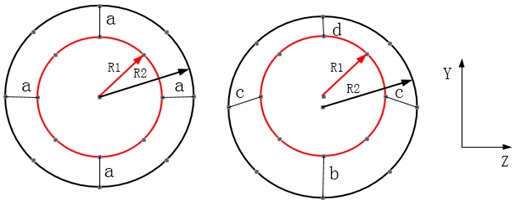Rotational inertia about the mass center ${I}_{xx}$, ${I}_{xz}$, ${I}_{yz}$, ${I}_{xy}$associated with the mass eccentricity were shown in Fig. 14. The structural parameters are associated with mass distribution, and therefore rotational inertia hold various values with different deviation of mass center, which can significantly affect the dynamics response of barrel.

Fig. 14. The value of a) ${I}_{xx}$, b) ${I}_{xy}$, c) ${I}_{xz}$ and d) ${I}_{yz}$ correlated to droop amount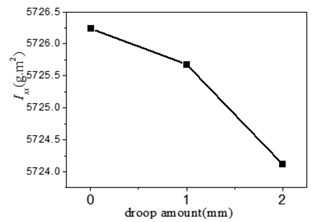a)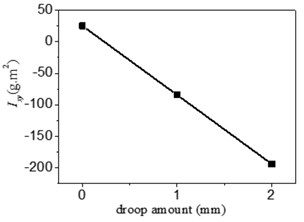b)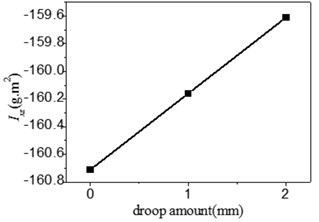c)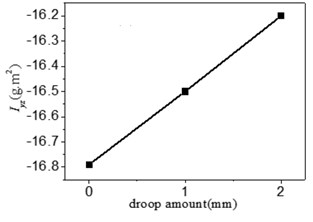d)

#### 4.4.1. Modal analysis

The modal analysis of structure can not only be used to determine the vibration characteristics of designed structure, intrinsic frequency and vibration mode, but also provides the fundamental support for other dynamics analysis. The transverse vibration of barrel is related to the stiffness and mass distribution and is composed of fundamental vibration and several high-order vibration components. The low-order vibration components with higher vibration amplitude and less frequency play critical roles in transverse vibration. Accordingly, in order to analyze the influence of mass eccentricity on the modal characteristics, the Lanczos method was adopted to perform the modal analysis and the first ten natural frequencies under different deviation conditions were extracted. We assume the results from axisymmetric condition as the standard value and the results are shown in Fig. 15.

Fig. 15. The comparison of natural frequencies for the given simulation conditions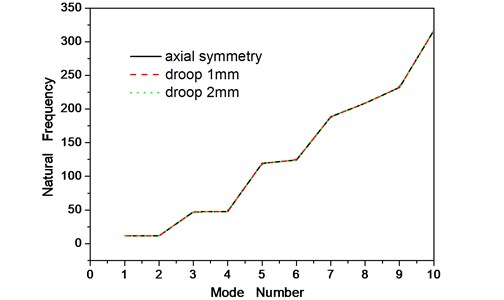Barrel droop leads to mass redistribution and influences the overall stiffness. Accordingly, as the mass center deviates, the overall mass matrix and stiffness matrix are updated, which ultimately brings different values of modal frequencies. From the obtained results, it is clear that natural frequencies of each mode decrease with the increasing value of barrel droop.

#### 4.4.2. Dynamics response

Additionally, with the aid of realization of radial loading of barrel with user subroutine, the firing process during interior ballistic course, with the two assumed mass eccentricity conditions, were performed to investigate the effect of mass eccentricity on dynamics response of barrel. Finally, associated computation results were obtained.

Fig. 16 and Fig. 17 shows the transverse displacement curves in horizontal and vertical directions, respectively. A similarity in variation trends for the given simulation conditions can be observed, while the magnitudes exhibit slight difference. Clearly, the presence of mass eccentricity produces more violent response than the axial symmetry condition, and the magnitudes for the 2 mm droop case are higher than the other two cases. More importantly, the muzzle vibration values at the instant when projectile exits muzzle increases with the increasing value of barrel droop. The absolute values for the 2 mm droop case are 0.00084 mm in horizontal direction and 0.61 mm in vertical direction, 2.4 % (0.00082 mm) and 8.2 % (0.56 mm) higher than the 1 mm droop case, and the same comparison results are 67.7 % (0.00027 mm) and 11.5 % (0.00054 mm) for the axial symmetry case. It can be concluded that the transverse vibration of barrel is influenced by mass distribution and larger mass eccentricity brings more violent results.

From the viewpoint of dynamic strength, the maximum equivalent stress curves in time domain were also plotted in Fig. 18 for comparison. The comparison results of dynamic strength are very similar to that of transverse displacement results, and the strength response is more violent when barrel droop value grows. For the 2 mm droop case, the second peak value which shows the extreme stress response is 665.8 MPa, approximately 3.0 % (645.5 MPa) higher than the 1 mm droop case and 7.6 % (615.5 MPa) than the axial symmetry case. Apparently, it is proposed that the mass eccentricity have significant effects on the dynamics response of barrel, which will be further investigated in our future work.

The above observations show that the occurrence of mass eccentricity could bring more violent dynamics response for barrel and influences muzzle vibration adversely especially at the instant when projectile exits muzzle, which indicates that the long-term used barrel with various degrees of wear and mass sagging should be replaced timely.

Fig. 16. The comparison of natural frequencies for the given simulation conditions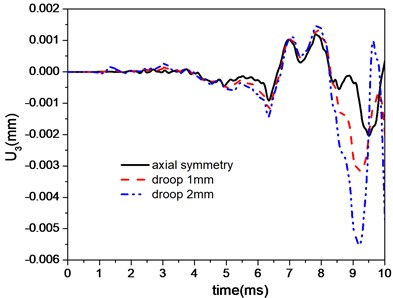Fig. 17. The muzzle displacement in vertical direction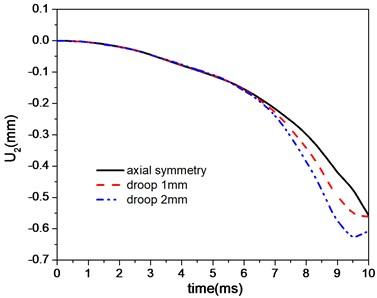Fig. 18. Stress peak distribution curves of barrel in time domain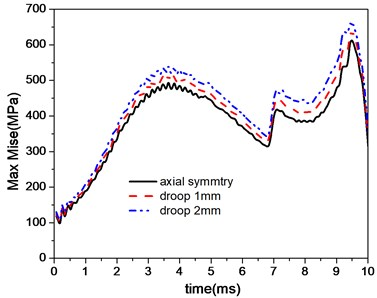#### 5. Conclusions

This paper presented an approach to realizing the dynamic loading process of propellant gas pressure on the tube inner wall. The dynamics response of barrel was investigated. Additionally, the effect of mass eccentricity of barrel on its dynamics response was studied under the realization of loading process. Based on the simulation results, the conclusions can be drawn as follows:

1) With the assistance of user-defined subroutine provided by main program, the dynamic loading process of the propellant gas pressure on the tube inner wall which is restricted by the motion position of projectile and the actual time was realized. This achievement can provide necessary means to model complete loading conditions for correlated numerical studies of artillery.

2) The comparison results in the time domain evidently show that the condition with the consideration of the radial effect of gas pressure which is more consistent with the actual loading condition brings more violent dynamics response, which implies that the radial effect of propellant gas is a non-negligible factor when simulating artillery firing process.

3) When the mass center of barrel deviates, the mass distribution influences the overall stiffness of barrel and generates less modal frequencies and more violent dynamics response. As the deviation increases, the dynamics response grows more violent, especially for the muzzle vibration displacement when the projectile passes muzzle. From the comparison, we can conclude that the dynamics response of barrel is more sensitive to the mass eccentricity, which indicates that the long-term used barrel with various degrees of wear and mass sagging should be replaced timely.

4) This work provides a comparatively perfect simulation environment that considers the radial effect of gas pressure,which makes valuable contributions to the cognition of artillery firing characteristics. However, the computation model still lacks rationality partially due to the negligence of the thermal effect including thermal loads. Future plans will focus on the thermal effect on the dynamics response of barrel and further development is ongoing to incorporate the velocity-dependent friction model into the Finite Element simulation.

#### Acknowledgements

The authors would like to acknowledge the funding from the National Natural Science Foundation of China (No. 11572158 and 51705253).

1. Gimm H. I., Cha K. U., Cho C. K. Characterizations of gun barrel vibrations of during firing based on shock response analysis and short-time Fourier transform. Journal of Mechanical Science and Technology, Vol. 26, Issue 5, 2012, p. 1463-1470. [Publisher]
2. Procházka S., Seman P., Vídeňka M. Deformation of the cannon barrel when projectile goes by muzzle. Proceedings of 9th Symposium on Weapon Systems, 2009, p. 43-49. [Search CrossRef]
3. Zhang X. B., Wang Y. Z. Analysis of dynamic characteristics for rarefaction wave gun during the launching. Journal of Applied Mechanics, Vol. 77, Issue 5, 2010, p. 1003-1013. [Publisher]
4. Yue Dong S., De Shi W. Study on vibration characteristics of barrel subjected to moving projectile considering inertia effect. Acta Armamentarii, Vol. 32, Issue 1, 2011, p. 414-419. [Search CrossRef]
5. Su Z. T., Xu D., Li X. W., Han Z. F. Finite-element time-history analysis for dynamic response of small-caliber guns with projectile-barrel coupling. Journal of Vibration and Shock, Vol. 23, 2012. [Search CrossRef]
6. Li Zhen, et al. Modeling and dynamic simulation on engraving process of rotating band into rifled barrel using three different numerical methods. Journal of Vibroengineering, Vol. 18, Issue 2, 2016, p. 768-780. [Search CrossRef]
7. Nadeem Ahmed, Brown Rod D., Hameed Amer Finite element modelling and simulation of gun dynamics using ANSYS. 10th International Conference on Computer Modeling and Simulation, 2008, p. 18-22. [Publisher]
8. Štiavnický M., Lisý P. Influence of barrel vibration on the barrel muzzle position at the moment when bullet exits barrel. Advances in Military Technology, Vol. 8, Issue 1, 2013, p. 89-102. [Search CrossRef]
9. Xu Yaofeng, Ding Hongmin, Xu Jian, Ning Bianfang Numerical analysis of influence of rifling structure of large caliber gun on moving of projectile with sliding driving band in bore. Acta Armamentarii, Vol. 37, Issue 11, 2016, p. 2148-2156. [Search CrossRef]
10. Siddiqui S. A., Golnaraghi M. F., Heppler G. R. Large free vibrations of a beam carrying a moving mass. International Journal of Non-linear Mechanics, Vol. 38, Issue 10, 2003, p. 1481-1493. [Publisher]
11. Siddiqui S. A. Q., Golnaraghi M. F., Heppler G. R. Dynamics of a flexible beam carrying a moving mass using perturbation, numerical and time-frequency analysis techniques. Journal of Sound and Vibration, Vol. 229, Issue 5, 2000, p. 1023-1055. [Publisher]
12. Ma D., Chen D., Wu S., et al. Dynamic experimental verification of void coalescence criteria. Materials Science and Engineering: A, Vol. 533, 2012, p. 96-106. [Publisher]
13. ABAQUS User’s Manual. Version 6.6, Hibbitt, Karlsson and Sorensen Inc, Michigan, 2006. [Search CrossRef]
14. Wu B., Zheng J., Tian Q. T., Zou Z. Q., Chen X. L., Zhang K. S. Friction and wear between rotating band and gun barrel during engraving process. Wear, Vol. 318, Issue 1, 2014, p. 106-13. [Publisher]
15. Bulman David N. The Effects of Barrel droop on Gun Barrel Response. School of Mechanical, Materials and Civil Engineering, RMCS, Shrivenham, Wiltshire, UK. [Search CrossRef]
16. Ahmed Brown N., January R. D. Modeling of dynamic interaction between gun system components during the recoil motion of the gun. International Design Engineering Technical Conferences and Computers and Information in Engineering Conference, 2009, p. 733-738. [Publisher]

#### Cited By

 Defence Technology David Leonhardt, Mark Garnich, Miljenko Lucic 2022